# How to Generate Random Decimal/Integer Numbers in Excel?

Random numbers are the random values, used to obtain some results. It can be used to perform simulations and to understand the pattern of different system components. It is useful when the user requires a large data set to evaluate some common feature. Decimal numbers are numbers that contain some fractional part. For example, ,1.2, and 3.5 are decimal numbers. While integer data contain numbers without fractional parts. An example of integer value is 1,2,5, and many other, that does not contain a decimal value.

## Example 1: To generate a random decimal number in Excel by using the predefined formula:

### Step 1

Consider the below-given data set. The provided table contains a column heading and some empty data rows.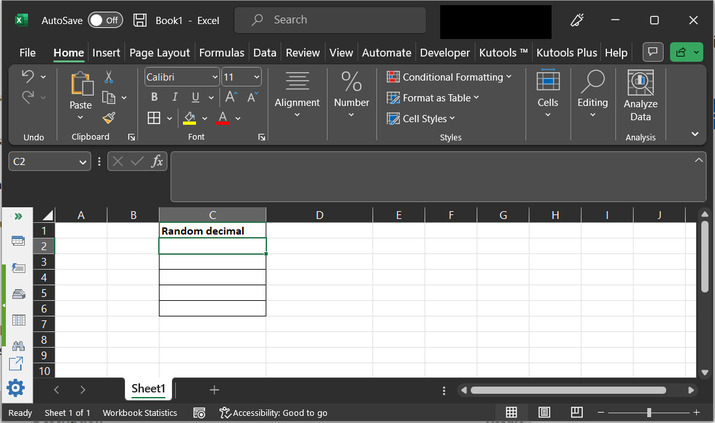### Step 2

In this example, the user is trained to use RAND() method. This method is used to generate a random number within Excel, without any need to write any formula or function. Simply call the method, with the range parameters to generate the random values.

Go to the C2 cell and type “=RAND()*100.00”. Consider depicted image −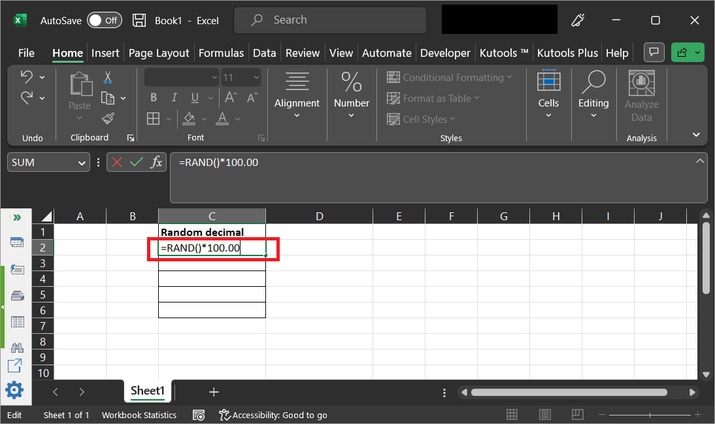### Step 3

After that press “Enter” key. This will display a random decimal value on sheet.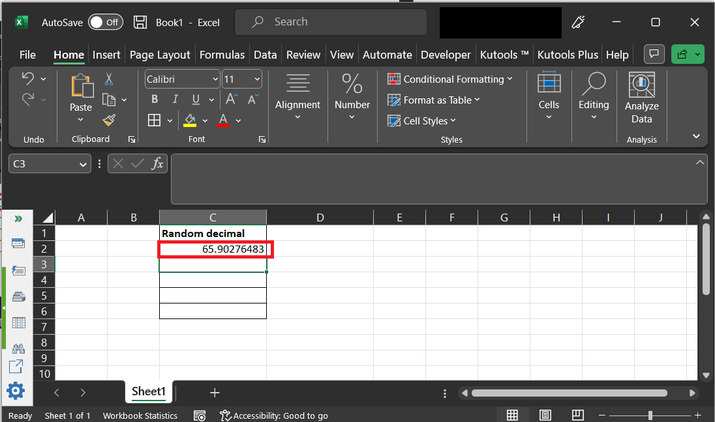## Example 2: To generate a random integer number in Excel by using the predefined formula:

### Step 1

Consider a worksheet, with a table that contains a column header “Random decimal”, with some empty data rows.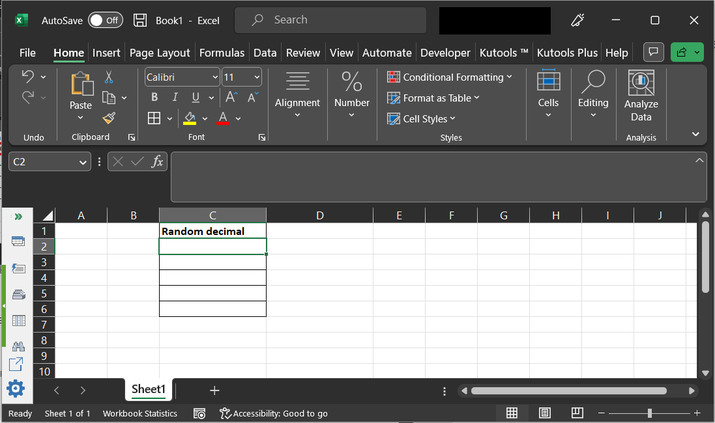### Step 2

Again, use RAND() method to generate the integer data. Simply go to the C2 column and type “=RAND()*100” as highlighted in the below image.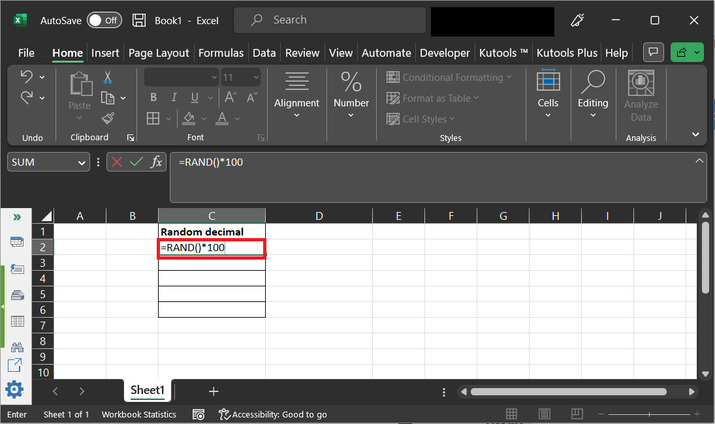### Step 3:

After typing the formula and press the “Enter” key. This will print the required random number on the sheet.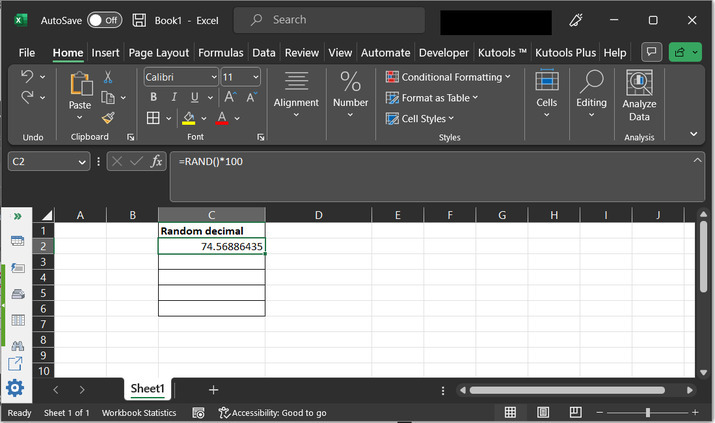### Step 4

Now, let’s round the obtained number as an integer value. To do so, go to the “Home” tab and then click on “Number” and select the “Decrease Decimal” option, as specified below: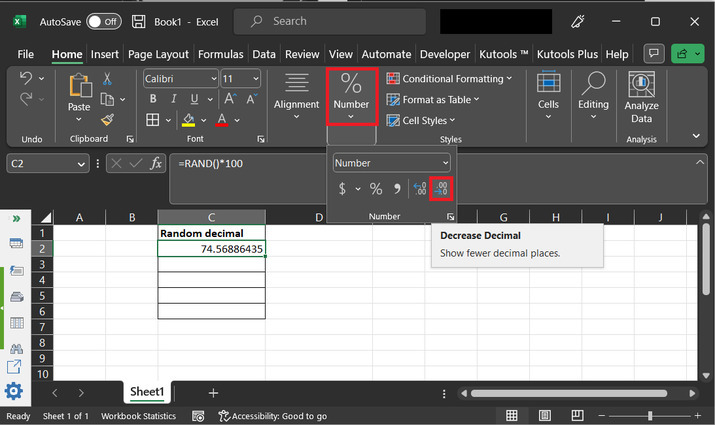### Step 5:

This will round the number to an integer value as specified below: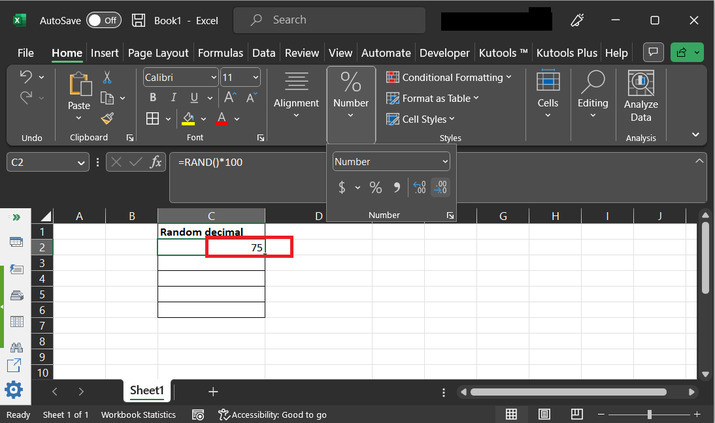## Example 3: To generate random integer number in Excel by using kutool:

### Step 1

Consider an empty worksheet. Open the “Kutools” option tab. Click on the “Editing” option, and after that select the “Insert” option under the “Editing” section. Finally choose “Insert Random Data”, as depicted below: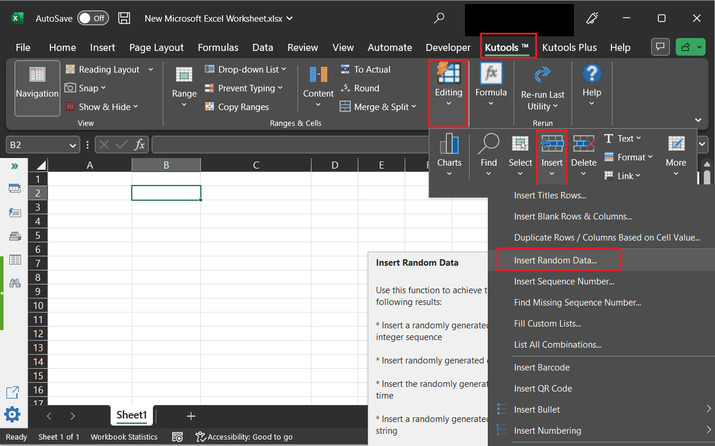### Step 2:

This will open the below depicted “Insert Random Data” dialog box to the user: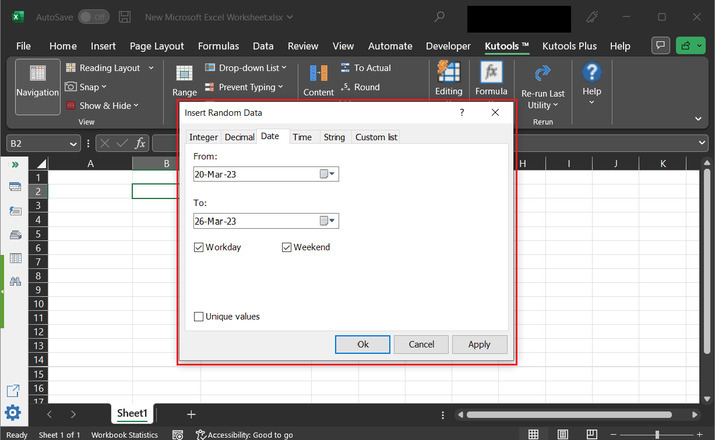### Step 3:

Click on the “Integer” option. As, here user wants to generate random integer values.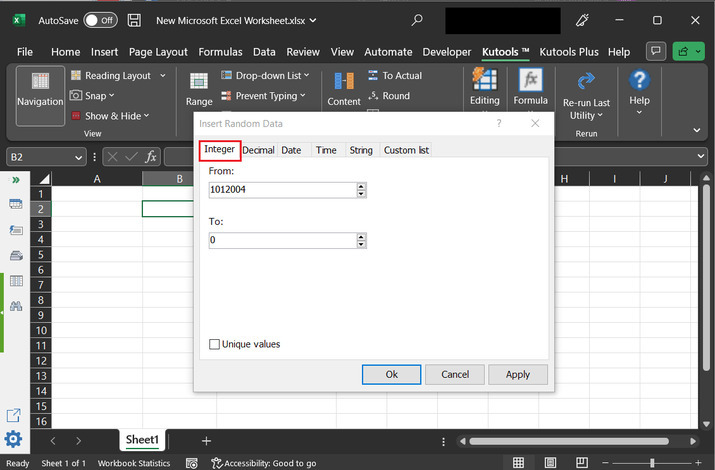### Step 4:

Add data to “From” and “To” labels, and click on the “Ok” button, as specified below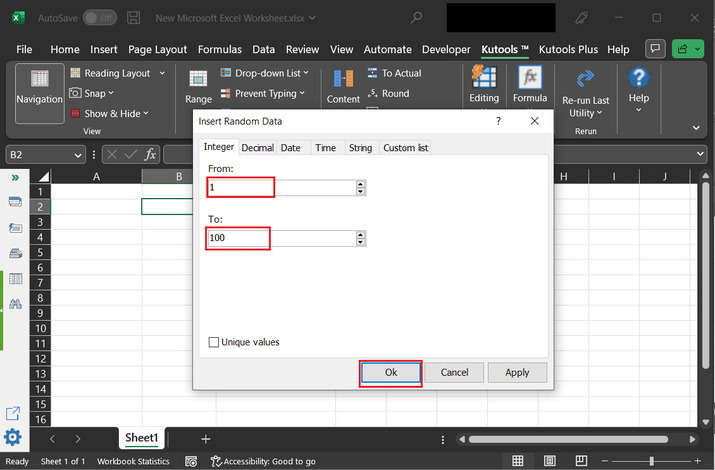### Step 5:

After the successful completion of the above step, A random integer value from the selected range will be printed automatically to the active cell. Consider a snapshot of the Excel sheet to understand the generated output.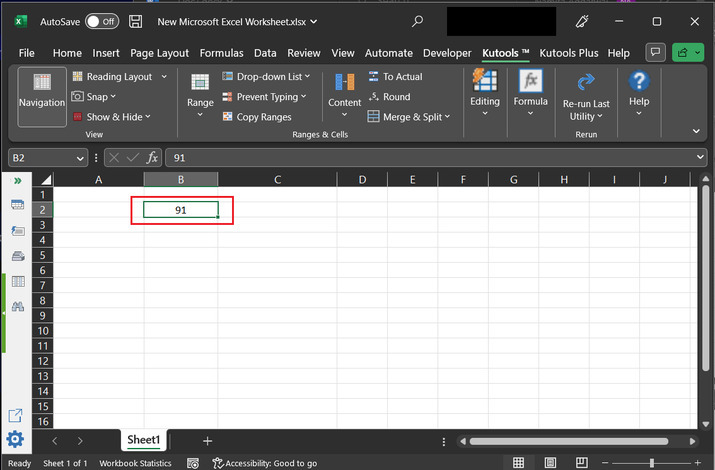## Example 4: To develop the random decimal number in Excel by using Kutool:

### Step 1:

Consider an empty worksheet. After that go to the “Kutools” option. Click on the “Editing” option, and after that select the “Insert” option from the “Editing” section. Finally, choose “Insert Random Data” as highlighted in the below image.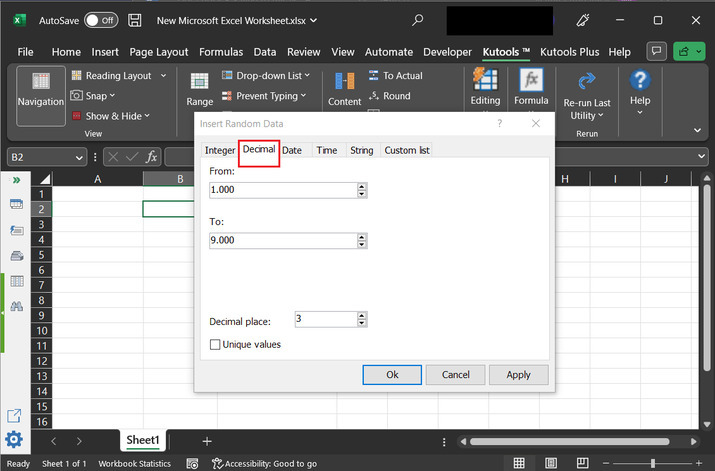### Step 2:

This will open the below depicted “Insert Random Data” dialog box to user:### Step 3

Click on “Decimal” tab. As, here user wants to generate random decimal values.### Step 4:

Add data to the “From” and “To” labels. This will act like the range. Here, the user can also able to select the possible decimal value. Let’s change the decimal value to 2 only. Finally, click on the “Ok” button, as specified below: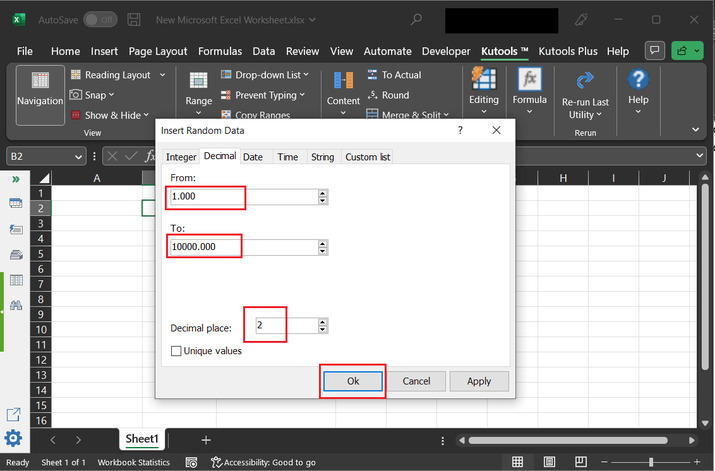### Step 5:

This will display a random decimal value to the active spreadsheet cell.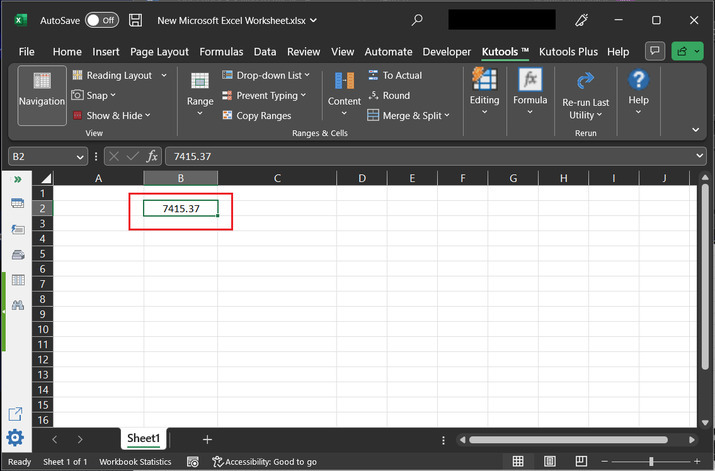## Conclusion:

This article provides four examples to generate random decimal and integer values. The first two examples are based on the excel predefined formula. While the other two examples are based on the use of kutools.

Updated on: 17-Apr-2023

122 Views